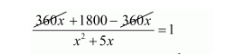# A train travels 360 km at a uniform speed.

Question:

A train travels 360 km at a uniform speed. If the speed had been 5 km/hr more, it would have taken 1 hour less for the same journey. Find the speed of the train.

Solution:

Let the original speed of train be $x \mathrm{~km} / \mathrm{hr}$. Then,

Increased speed of the train $=(x+5) \mathrm{km} / \mathrm{hr}$

Time taken by the train under usual speed to cover $360 \mathrm{~km}=\frac{360}{x} \mathrm{hr}$

Time taken by the train under increased speed to cover $360 \mathrm{~km}=\frac{360}{(x+5)} \mathrm{hr}$

Therefore,

$\frac{360}{x}-\frac{360}{(x+5)}=1$

$\frac{\{360(x+5)-360 x\}}{x(x+5)}=1$

$\frac{360 x+1800-360 x}{x^{2}+5 x}=1$$1800=x^{2}+5 x$

$x^{2}+5 x-1800=0$

$x^{2}+5 x-1800=0$

$x^{2}-40 x+45 x-1800=0$

$x(x-40)+45(x-40)=0$

$(x-40)(x+45)=0$

So, either

$(x-40)=0$

$x=40$

Or

$(x+45)=0$

$x=-45$

But, the speed of the train can never be negative.

Hence, the original speed of train is $x=40 \mathrm{~km} / \mathrm{hr}$